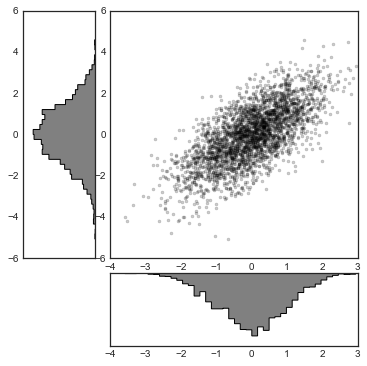# 8.11 多个子图

``````%matplotlib inline
import matplotlib.pyplot as plt
plt.style.use('seaborn-white')
import numpy as np
``````

## `plt.axes`：手动创建子图

``````ax1 = plt.axes()  # 标准轴域
ax2 = plt.axes([0.65, 0.65, 0.2, 0.2])
``````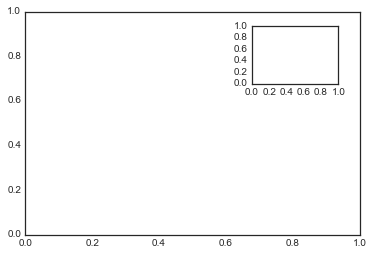``````fig = plt.figure()
ax1 = fig.add_axes([0.1, 0.5, 0.8, 0.4],
xticklabels=[], ylim=(-1.2, 1.2))
ax2 = fig.add_axes([0.1, 0.1, 0.8, 0.4],
ylim=(-1.2, 1.2))

x = np.linspace(0, 10)
ax1.plot(np.sin(x))
ax2.plot(np.cos(x));
``````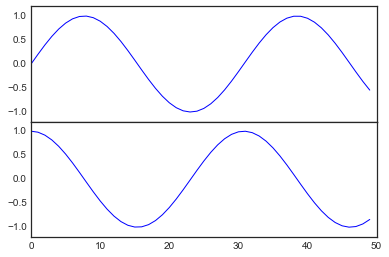## `plt.subplot`：子图的简单网格

``````for i in range(1, 7):
plt.subplot(2, 3, i)
plt.text(0.5, 0.5, str((2, 3, i)),
fontsize=18, ha='center')
``````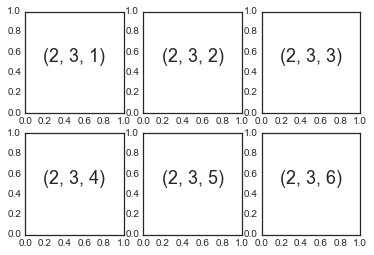``````fig = plt.figure()
for i in range(1, 7):
ax.text(0.5, 0.5, str((2, 3, i)),
fontsize=18, ha='center')
``````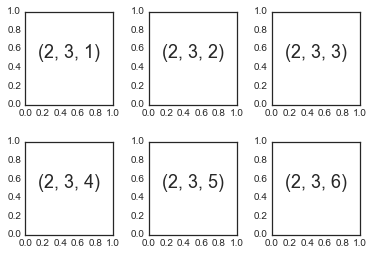## `plt.subplots`：一次创建整个网格

``````fig, ax = plt.subplots(2, 3, sharex='col', sharey='row')
``````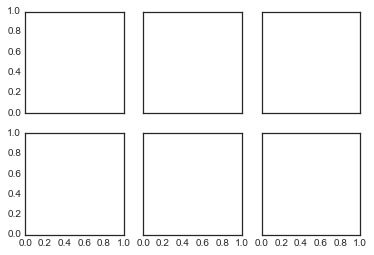``````# ax 是二维数组，由 [row, col] 索引
for i in range(2):
for j in range(3):
ax[i, j].text(0.5, 0.5, str((i, j)),
fontsize=18, ha='center')
fig
``````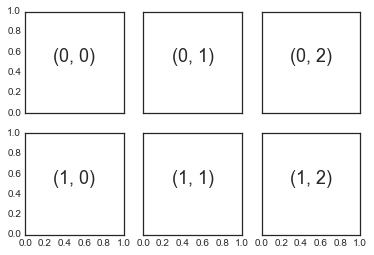`plt.subplot()`相比，`plt.subplots()`与 Python 传统的基于 0 的索引更加一致。

## `plt.GridSpec`：更加复杂的排列

``````grid = plt.GridSpec(2, 3, wspace=0.4, hspace=0.3)
``````

``````plt.subplot(grid[0, 0])
plt.subplot(grid[0, 1:])
plt.subplot(grid[1, :2])
plt.subplot(grid[1, 2]);
``````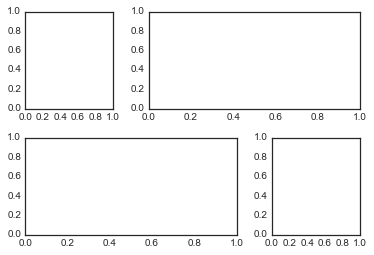``````# 创建一些正态分布的数据
mean = [0, 0]
cov = [[1, 1], [1, 2]]
x, y = np.random.multivariate_normal(mean, cov, 3000).T

# 使用 gridspec 建立轴域
fig = plt.figure(figsize=(6, 6))
grid = plt.GridSpec(4, 4, hspace=0.2, wspace=0.2)
y_hist = fig.add_subplot(grid[:-1, 0], xticklabels=[], sharey=main_ax)
x_hist = fig.add_subplot(grid[-1, 1:], yticklabels=[], sharex=main_ax)

# 主要轴域上的散点图
main_ax.plot(x, y, 'ok', markersize=3, alpha=0.2)

# 附加轴域上的直方图
x_hist.hist(x, 40, histtype='stepfilled',
orientation='vertical', color='gray')
x_hist.invert_yaxis()

y_hist.hist(y, 40, histtype='stepfilled',
orientation='horizontal', color='gray')
y_hist.invert_xaxis()
``````